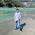Monday, December 12, 2016

ECG basics

So basically, ECG is  an electrical representation of heart activity on ECG paper.

Here are some important postulates of ECG paper:

The needle of galvanometer travels 300 big squares in 1 minute or 60 seconds.

That makes 0.2 seconds for each big square.

In turn each big square is divided into 5 small boxes /squares.

So we get each small box counting about
0.04 seconds.

So let's review all calculations:

1 Big square = 0.2 seconds.
1 small square = 0.04 seconds.

Each P wave travels about 2 and 1/2 small  squares making upto 0.1 second.

The segment PQ (Sometimes we use PR) again takes 2 and 1/2 small squares making upto 0.1 second.

QRS complex used another 2 and 1/2  small squares making another 0.1 second.

While S-T segments uses 10 small squares forming using 0.4 seconds.

Note: We normally consider 2 and 1/2 small squares but there can be 2 or 3 small squares.

~Ojas

1.So total comes to be 0.7 seconds, it should be 0.8 no?

1.PQ segment is considered approximately 2 and 1/2.Its variable that's why.

2.We need to consider ST interval not ST segment. So that adds another 0.1 making it 0.8s

This is express yourself space. Where you type create something beautiful! <3
Wondering what do I write? Well..
Tell us something you know better. You are a brilliant mind. Yes, you are! ^__^
Ask about something you don't understand @_@?
Compliment... Say something nice! =D
Be a good critic and correct us if something went wrong :|
Go ahead. Comment all you like here! (: